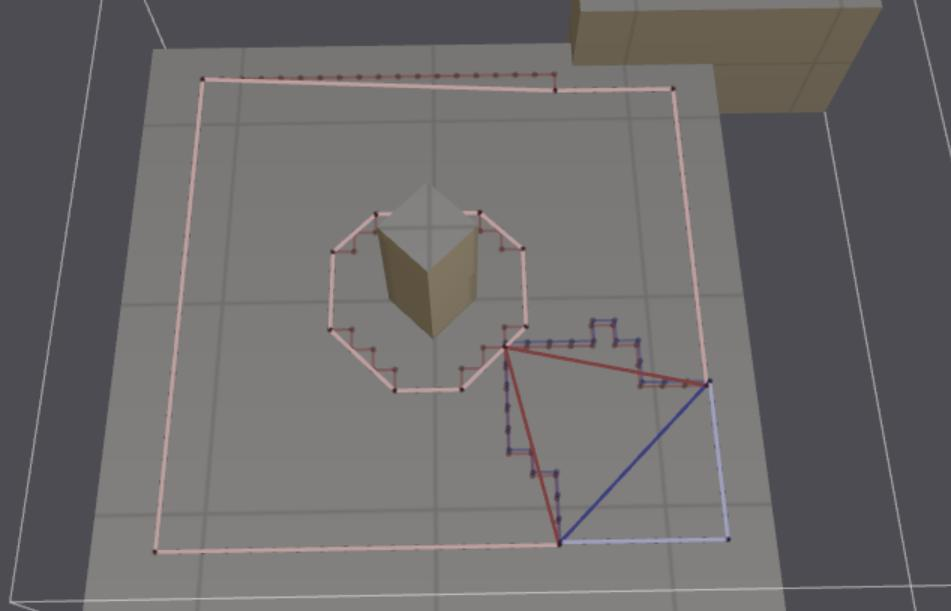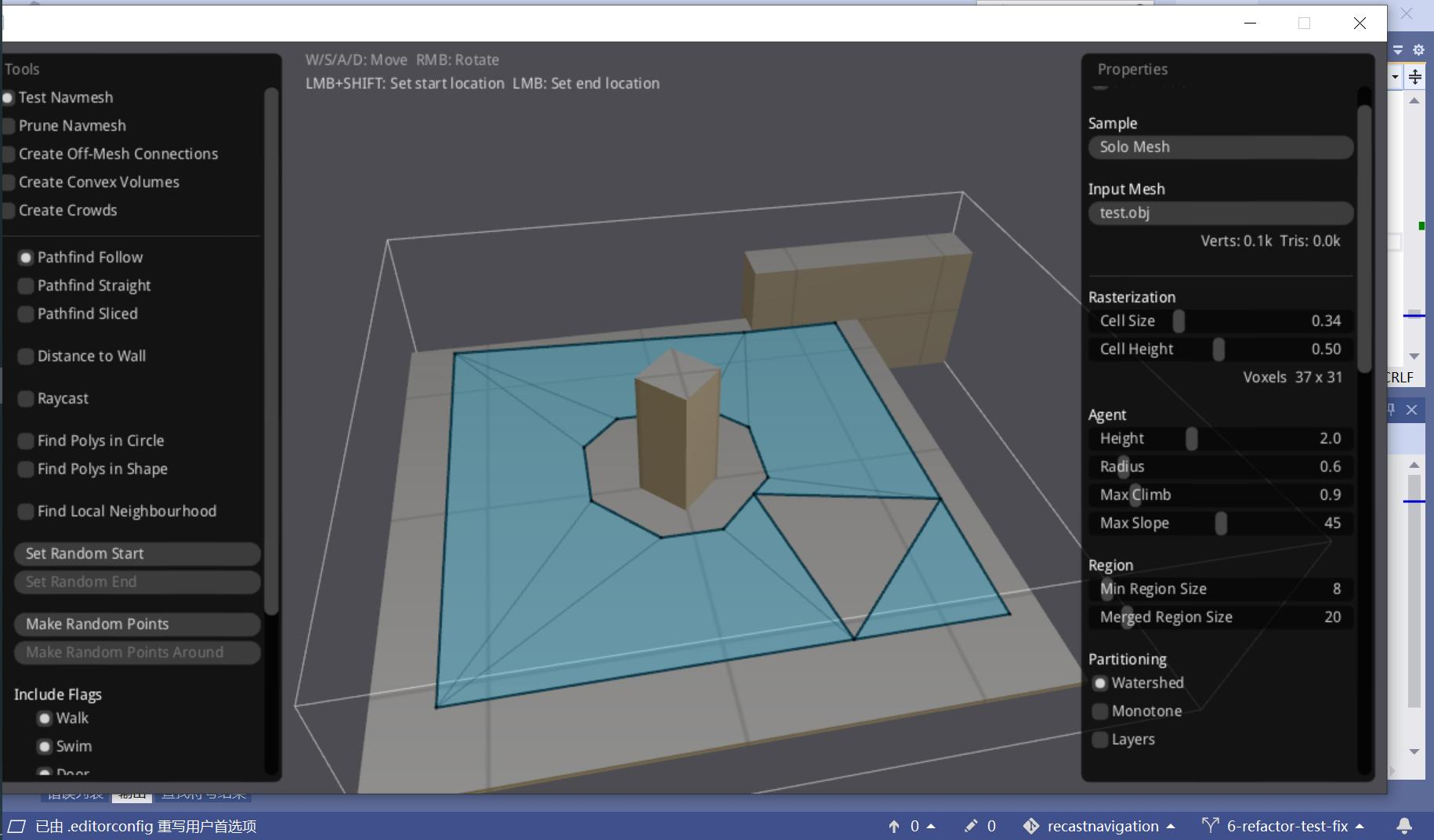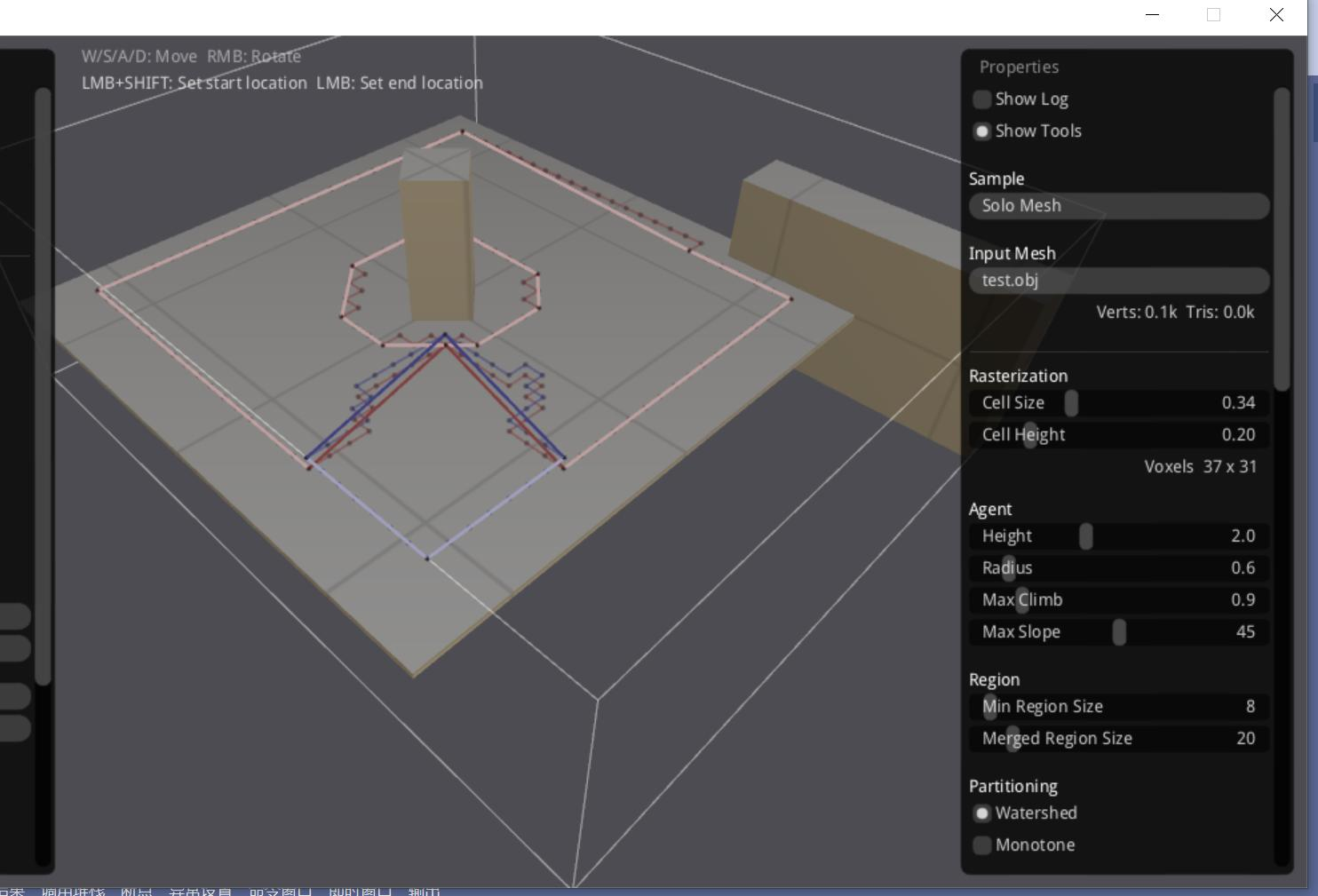# 金庆的专栏

C++博客 :: 首页 :: 新随笔 :: 联系 :: 聚合:: 管理 ::423 随笔 :: 0 文章 :: 454 评论 :: 0 Trackbacks

# Fix navmesh countour

(Jin Qing's Column, Jan., 2023)
After changing some parameters of watershed region partition algorithm,
my test mesh generated an odd region shape, which caused a wrong contour.
Wrong contour:Wrong navmesh:There are 2 regions, the big region with red contour forms a C shape,
which is closed at one point.
The error is caused by the closing point, which should be a vertex in the simplified contour
of the small blue region but not.
In simplifyContour(), the closing point is incorrectly regarded as the edge point between 2 regions,
and is skipped, but it is accurately a vertex among 3 regions and must be a contour vertex.
To fix the problem, each vertex of the raw contour should count the regions around the vertex.
If the regions count is 3 or 4, then it must be a contour vertex of the simplified contour.
Fixed contour:Code:
```
// Returns the regions count of the contour vertex's 4 cells.
// The contour vertex is the front-right of the current span.
// This vertex must be the simplified contour's vertex.
// The non-region(0) is count as a region.
//
// reg is 4 region IDs of 4 cells in clockwise order: 0-current, 1-dir, 2-diagonal, 3-dirp
//
// Diagonal cells are not connected, so these 4 cells have regions count 4,
//   because region a and b are separated from each other:
//   ```
//   a|b
//   -+-
//   b|a
// ```
size_t getRegionsCount(const rcRegionId reg)
{
size_t diffCount = 0;
for (size_t i = 0, j = 3; i < 4; j = i++)
{
if (reg[i] != reg[j])
++diffCount;
}
assert(diffCount <= 4);
assert(diffCount != 1);
if (0 == diffCount)
return 1;
return diffCount;
}
void testGetRegionsCount()
{
assert(1 == getRegionsCount4(1, 1, 1, 1));
assert(2 == getRegionsCount4(1, 0, 0, 0));
assert(2 == getRegionsCount4(1, 1, 0, 0));
assert(3 == getRegionsCount4(0, 1, 2, 0));
assert(4 == getRegionsCount4(0, 1, 2, 3));
assert(4 == getRegionsCount4(1, 0, 1, 0));
assert(4 == getRegionsCount4(1, 0, 2, 0));
}
```

test.obj: /Files/jinq0123/test.zip
posted on 2023-01-01 10:37 金庆 阅读(134) 评论(0)  编辑 收藏 引用